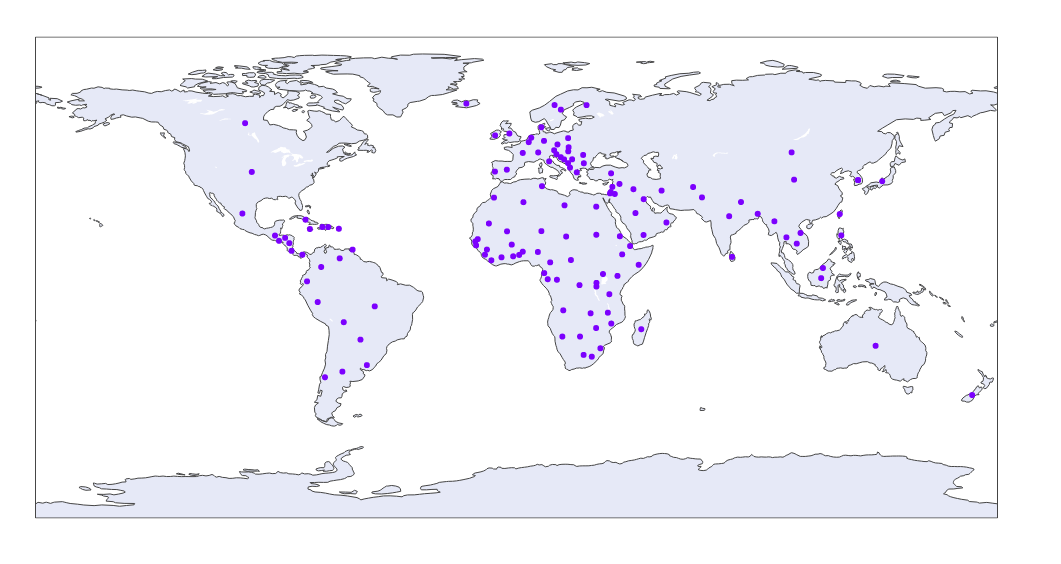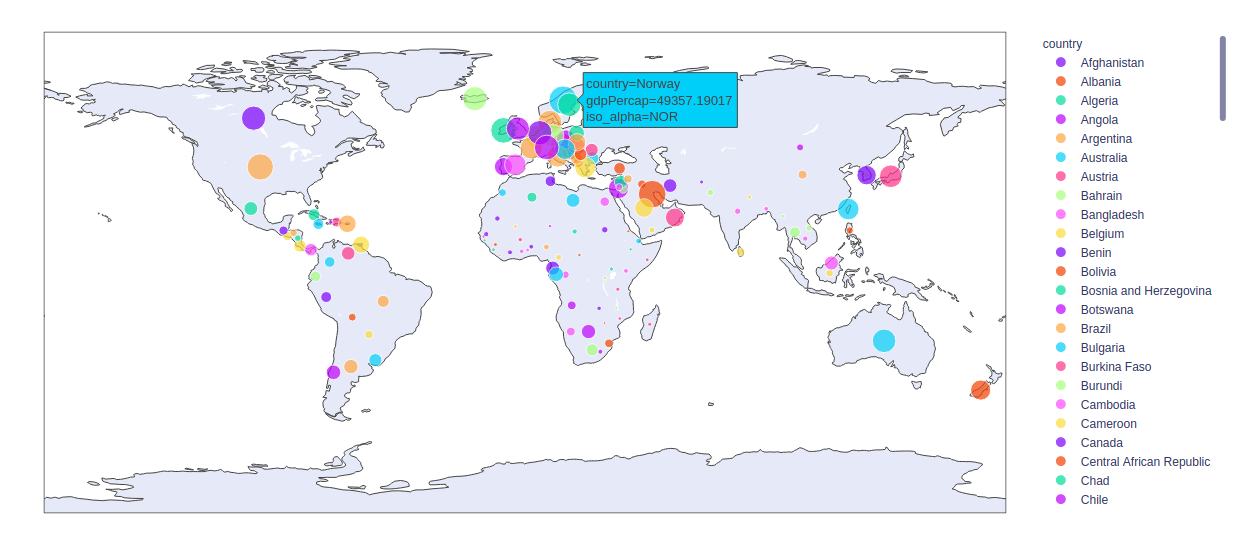Open In App

# plotly.express.scatter_geo() function in Python

Plotly library of Python can be very useful for data visualization and understanding the data simply and easily. Plotly graph objects are a high-level interface to plotly which are easy to use.

## plotly.express.scatter_geo() function

This function is used to plot geographical data onto the maps.

Syntax: plotly.express.scatter_geo(data_frame=None, lat=None, lon=None, locations=None, locationmode=None, color=None, text=None, hover_name=None, hover_data=None, custom_data=None, size=None, title=None, template=None, width=None, height=None)

Parameters:

data_frame: DataFrame or array-like or dict needs to be passed for column names.

lat: This parameter is used to position marks according to latitude on a map.

lon: This parameter is used to position marks according to longitude on a map.

locations: This parameter is interpreted according to locationmode and mapped to longitude/latitude.

locationmode: This parameter determines the set of locations used to match entries in locations to regions on the map.

color: This parameters assign color to marks.

size: This parameter is used to assign mark sizes. It is either a name of a column in data_frame, or a pandas Series or array_like object.

title: This parameter sets the title of the figure.

width: This parameter sets the width of the figure

height: This parameter sets the height of the figure.

Example 1:

## Python3

 `import` `plotly.express as px`` ` ` ` `df ``=` `px.data.gapminder().query(``"year == 2007"``)`` ` `plot ``=` `px.scatter_geo(df, locations``=``"iso_alpha"``)``plot.show()`

Output:Example 2: Using the size and color argument

## Python3

 `import` `plotly.express as px`` ` ` ` `df ``=` `px.data.gapminder().query(``"year == 2007"``)`` ` `plot ``=` `px.scatter_geo(df, locations``=``"iso_alpha"``,``                      ``size``=``"gdpPercap"``,``                      ``color ``=` `"country"``)``plot.show()`

Output: